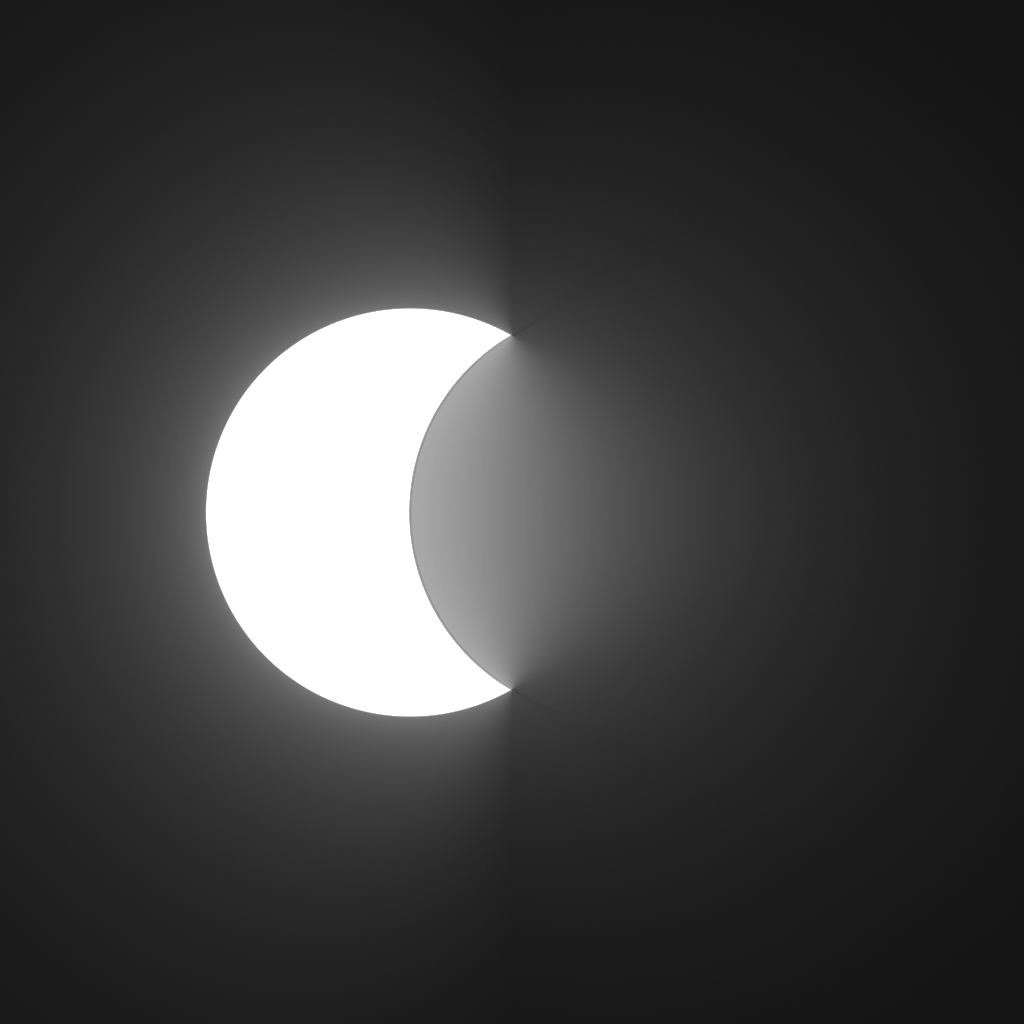## 1. 场景函数

float trace(float ox, float oy, float dx, float dy) {
float t = 0.0f;
for (int i = 0; i < MAX_STEP && t < MAX_DISTANCE; i++) {
float sd = circleSDF(ox + dx * t, oy + dy * t, /*...*/); // SDF
if (sd < EPSILON)
return 2.0f; // 自发光
t += sd;
}
return 0.0f;
}

typedef struct { float sd, emissive; } Result;

Result scene(float x, float y) {
Result r = { circleSDF(x, y, 0.5f, 0.5f, 0.1f), 2.0f };
return r;
}

float trace(float ox, float oy, float dx, float dy) {
float t = 0.0f;
for (int i = 0; i < MAX_STEP && t < MAX_DISTANCE; i++) {
Result r = scene(ox + dx * t, oy + dy * t); // <-
if (r.sd < EPSILON)                         // <-
return r.emissive;                      // <-
t += r.sd;                                  // <-
}
return 0.0f;
}

## 2. 加入多个形状Result unionOp(Result a, Result b) {
return a.sd < b.sd ? a : b;
}

Result scene(float x, float y) {
Result r1 = { circleSDF(x, y, 0.3f, 0.3f, 0.10f), 2.0f };
Result r2 = { circleSDF(x, y, 0.3f, 0.7f, 0.05f), 0.8f };
Result r3 = { circleSDF(x, y, 0.7f, 0.5f, 0.10f), 0.0f };
return unionOp(unionOp(r1, r2), r3);
}

## 3. 构造实体几何Result unionOp(Result a, Result b) {
return a.sd < b.sd ? a : b;
}

Result intersectOp(Result a, Result b) {
Result r = a.sd > b.sd ? b : a;
r.sd = a.sd > b.sd ? a.sd : b.sd;
return r;
}

Result subtractOp(Result a, Result b) {
Result r = a;
r.sd = (a.sd > -b.sd) ? a.sd : -b.sd;
return r;
}

Result scene(float x, float y) {
Result a = { circleSDF(x, y, 0.4f, 0.5f, 0.20f), 1.0f };
Result b = { circleSDF(x, y, 0.6f, 0.5f, 0.20f), 0.8f };
return unionOp(a, b);
// return intersectOp(a, b);
// return subtractOp(a, b);
// return subtractOp(b, a);
}